# Per Capita GDP by US State, First Quarter 2020

Jul 22, 2020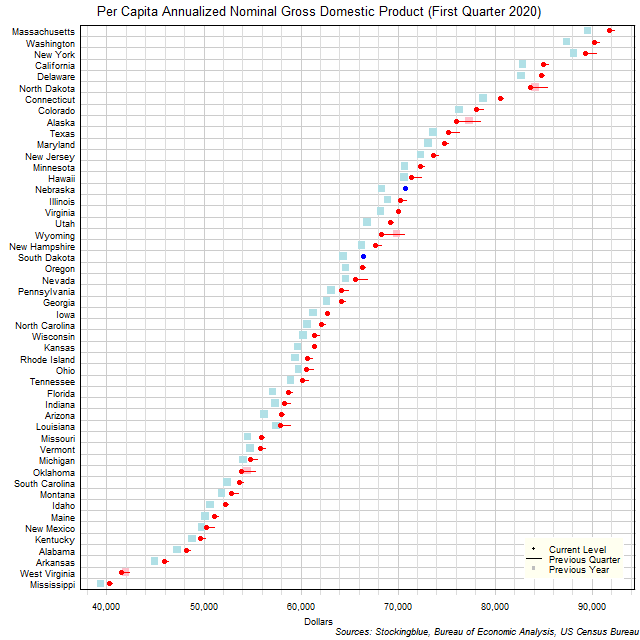The chart above shows the per capita annualized nominal gross domestic product (GDP) in each US state as of the first quarter of 2020 in dollars, the change from the previous quarter, and the GDP one year prior.  Nebraska and South Dakota were the only states to experience a gain in per capita GDP over the past quarter.

# EU and US GDP by Region, First Quarter 2020

Jul 21, 2020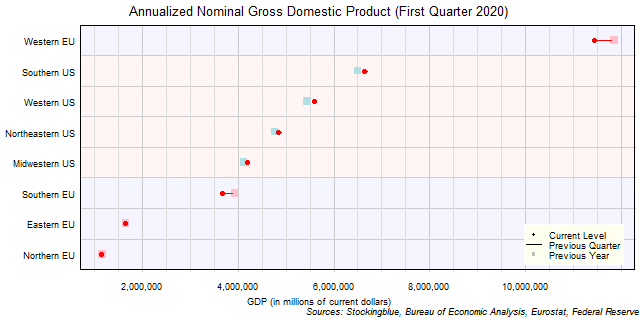The chart above shows the annualized nominal gross domestic product (GDP) in each EU and US region as of the first quarter of 2020 in millions of dollars, the change from the previous quarter, and the GDP one year prior.  The Western EU is the only EU region that has a larger GDP than any US region.

# EU GDP by Region, First Quarter 2020

Jul 20, 2020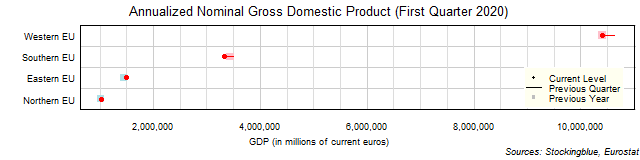The chart above shows the annualized nominal gross domestic product (GDP) in each EU region as of the first quarter of 2020 in millions of euros, the change from the previous quarter, and the GDP one year prior.  The Eastern and Northern EU were the only regions to see growth over the past year.

# US GDP by Region, First Quarter 2020

Jul 17, 2020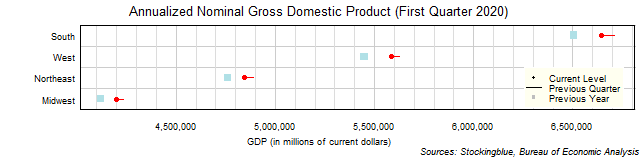The chart above shows the annualized nominal gross domestic product (GDP) in each US region as of the first quarter of 2020 in millions of dollars, the change from the previous quarter, and the GDP one year prior.  Every single region's economy contracted over the past quarter and grew over the past year.

# EU and US GDP by State, First Quarter 2020

Jul 16, 2020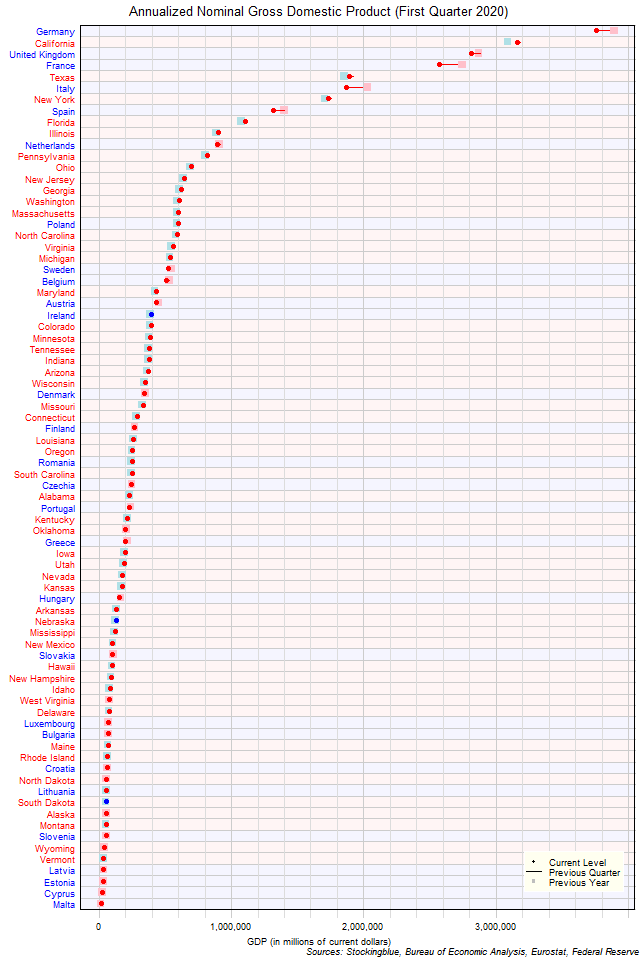The chart above shows the annualized nominal gross domestic product (GDP) in each EU and US state as of the first quarter of 2020 in millions of US dollars, the change from the previous quarter, and the GDP one year prior.  Only four states in both the EU and the US (three of which are from the EU) have an annualized GDP of over \$2 trillion.

# EU GDP by State, First Quarter 2020

Jul 15, 2020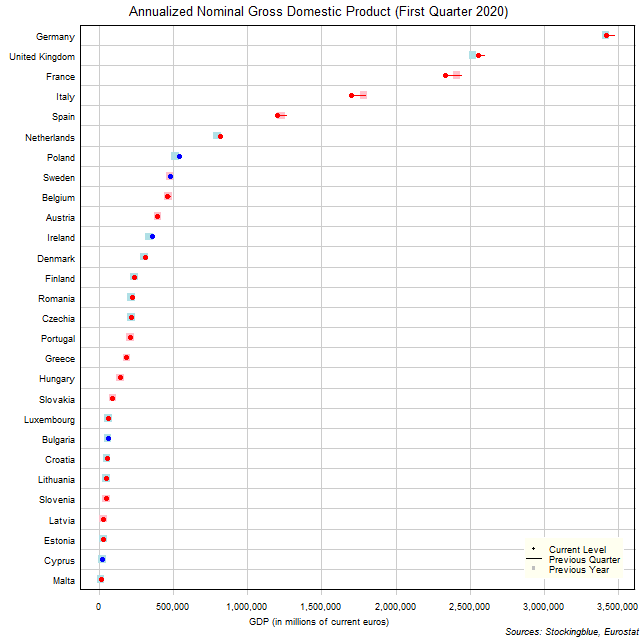The chart above shows the annualized nominal gross domestic product (GDP) in each EU state as of the first quarter of 2020 in millions of euros, the change from the previous quarter, and the GDP one year prior.  Poland, Sweden, Ireland, Bulgaria, and Cyprus are the only states to see a rise from the previous quarter.

# US GDP by State, First Quarter 2020

Jul 14, 2020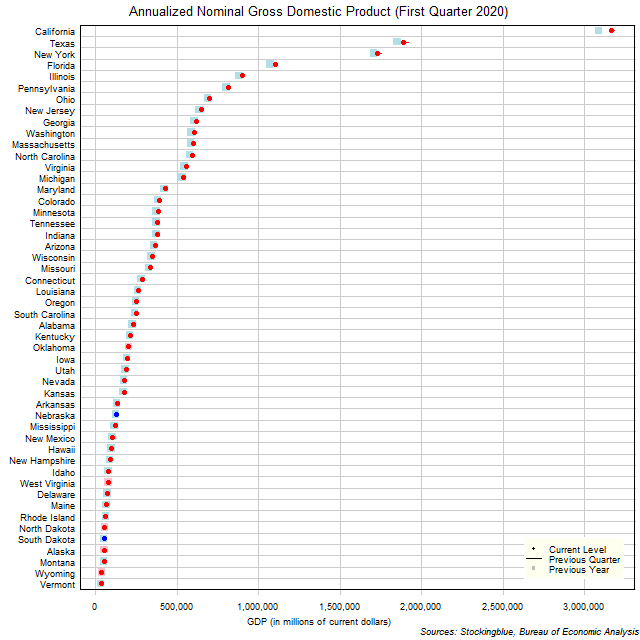The chart above shows the annualized nominal gross domestic product (GDP) in each US state as of the first quarter of 2020 in millions of dollars, the change from the previous quarter, and the GDP one year prior.  Nebraska and South Dakota were the only states to see an increase in GDP over the previous quarter.

# Long-Term EU and US GDP Growth Rate by Region, Fourth Quarter 2019

Jul 1, 2020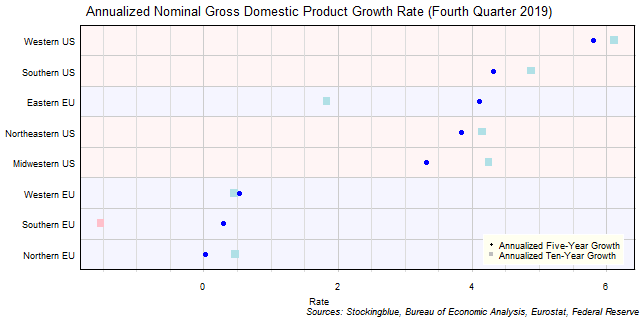The chart above shows the annualized nominal gross domestic product (GDP) growth rate when priced in US dollars in each EU and US region over the past five years as of the fourth quarter of 2019 and the growth over the past ten years.  All negative growth rates in the EU are attributed to currency rate fluctuations.

# Long-Term EU GDP Growth Rate by Region, Fourth Quarter 2019

Jun 30, 2020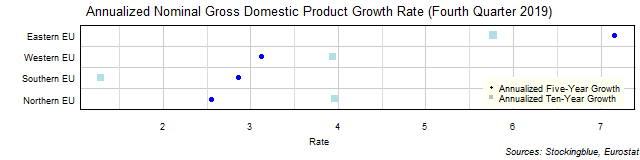The chart above shows the annualized nominal gross domestic product (GDP) growth rate in each EU region over the past five years as of the fourth quarter of 2019 and the growth rate over the past ten years.  The Western and Northern EU had a slower growth rate over the past five years than they had over the past ten years.

# Long-Term US GDP Growth Rate by Region, Fourth Quarter 2019

Jun 29, 2020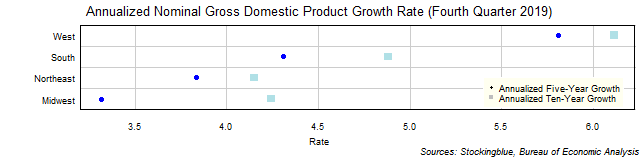The chart above shows the annualized nominal gross domestic product (GDP) growth rate in each US region over the past five years as of the fourth quarter of 2019 and the growth rate over the past ten years.  All four regions grew over both time periods.